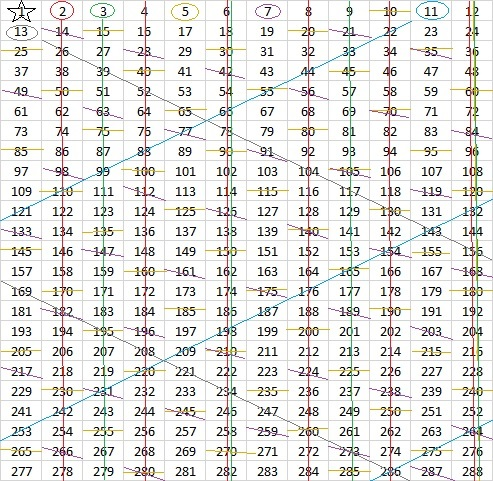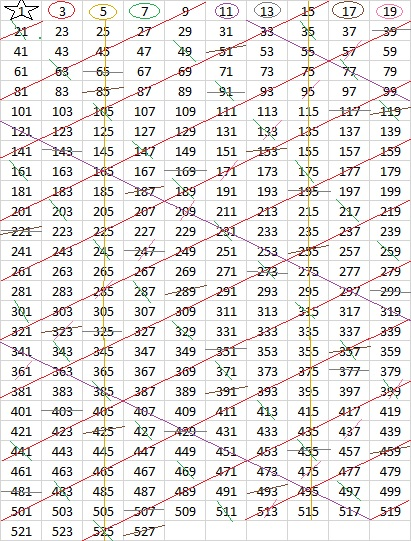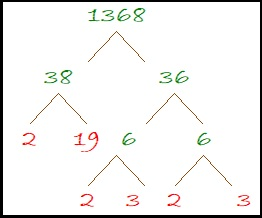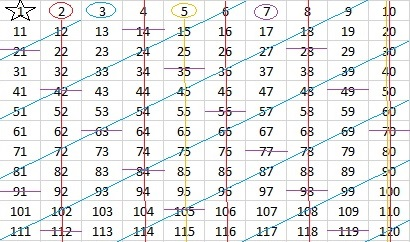# 1368 Playing with the Sieve of Eratosthenes

What if we didn’t use ten numbers across for our Sieve of Eratosthenes?

For example, 36 × 38 = 1368. We could make a Sieve of Eratosthenes writing 36 numbers across the grid and 38 numbers down. The last number on the grid would be 1368, and we could find all the prime numbers less than 1369 (which is 17²) by crossing out all the multiples of the prime numbers that appear on the top row. The trouble is that 36 numbers across makes a very big grid. Crossing out multiples of 2, 3, 5, and 7 will be very quick, but crossing out all the multiples of 11, 13, 17, 19, 23, 29, and 31 will not be so fun.

Grids that make use of the fact that (n-1)(n+1) = n²-1 can always give us a perfect rectangle and we will only need to cross out the multiples of the prime numbers in the top row to find ALL the prime numbers in any (n-1)×(n+1) list of numbers.

Here’s a 7 × 9 grid:Since it was 7 across, it was very easy to cross out all the multiples of 7. The multiples of 2 and 3 weren’t too difficult to find either, but the pattern for the multiples of 5 was not quite as nice. Fortunately, it is easy to spot those multiples, no matter how big a number they are.

Still, the first prime number on the second row is 11, so we should be able to go almost up to 11² = 121 on our grid:I couldn’t fit 120 on the grid without ruining the rectangle, but here’s a grid using 12 numbers across. Since 12 × 14 = 168 which is one less than 13², we can find all the prime numbers in the list simply by crossing out the multiples of the prime numbers in the top row.But the next number, 13, is only one number more than 12, and all of its multiples are staring at me making me feel very uncomfortable. It will be very easy to cross out all of the multiples of 13. That means we can extend the list of numbers to one less than the next prime number squared, which is 289 – 1 = 288. This time we get a perfect rectangle because 288 is also a multiple of 12:All of the circled numbers in the top row and every number that has not been crossed out below the top row are prime numbers.

Someone long ago figured out that if we make the grid six numbers across, all the prime numbers except 2 and 3 will appear in the same two columns, no matter how long the grid is:Every prime number greater than 3 is either one less or one more than a multiple of 6.

Since we always cross out the multiples of 2 anyway, what would happen if we didn’t include them in the grid at all?

Here is a grid with ten numbers across, but only odd numbers are included. Because 5 is a factor of 10, it is very easy to cross out all of the 5’s. Also, since 9 is one less than 10 and 11 is one more than 10, it is also easy to cross out all the multiples of 3 and 11. Crossing out the 7’s and the 19’s wasn’t too bad, either, but the 13’s and 17’s were not as fun.In my next post, I will share my favorite size of grid and the method I use to find all of the prime numbers on it. No prime numbers get circled in my method.

Some of the numbers in the grids had several lines through them.
If we made the 36 × 38 grid I mentioned at the beginning of the post, how many lines would 1368 have going through it?  After all, 1368 has 24 factors. What do you think?

Only three lines. One each for its prime factors, 2, 3 and 19.

Here’s more about the number 1368:

• 1368 is a composite number.
• Prime factorization: 1368 = 2 × 2 × 2 × 3 × 3 × 19, which can be written 1368 = 2³ × 3² × 19
• 1368 has at least one exponent greater than 1 in its prime factorization so √1368 can be simplified. Taking the factor pair from the factor pair table below with the largest square number factor, we get √1368 = (√36)(√38) = 6√38
• The exponents in the prime factorization are 3, 2, and 1. Adding one to each exponent and multiplying we get (3 + 1)(2 + 1)(1 + 1) = 4 × 3 × 2 = 24. Therefore 1368 has exactly 24 factors.
• The factors of 1368 are outlined with their factor pair partners in the graphic below.Here’s one of the MANY possible factor trees for 1368:# Finding Primes Like 1367

Ancient Greek Eratosthenes had a method of finding prime numbers. We call it the Sieve of Eratosthenes. You can use this method if you make a list of numbers, circle the first available prime number, cross out all of its multiples on the chart and repeat tat procedure over and over again. The next number that has not been crossed out is the next prime number. Numbers that get crossed out are composite numbers, and they can always be expressed as the product of prime numbers.

Many teachers have given their students a 100 chart to help them find the twenty-five prime numbers less than 100.If the number 1 were a prime number, we would have to cross out all of its multiples, and that would make 1 be the only prime number!?? That would be an unacceptable conclusion. It turns out that 1 cannot be either prime or composite. Perhaps you will want to put a star around it.

Here is a 100 chart with the multiples of 2, 3, 5, and 7 crossed out.Since 10 is a multiple of 2 and 5, it was easy to cross out their multiples. 9, a multiple of 3, is one less than 10, so 3’s multiples were also easy to cross out. Crossing out all the multiples of 7 is a little bit tedious at first, but you can even find a pattern for them as well.

Notice that the distance between multiples of any given prime is that prime number whether you count horizontally or vertically. The circled numbers AND all the numbers not crossed out on the chart are prime numbers.

This Sieve of Eratosthenes is a powerful method for finding prime numbers, but some of its power is lost when just a 100 chart is used. For pretty much the same amount of work, we could have used the list of numbers in a 10 × 12 chart because 10 × 12 = 120 which is one less than the next prime number squared, 11² = 121.But wait a minute! 11 is one more than 10 so its multiples would be SO easy to cross out. Let’s make the chart go to one less than 168 which is one less than 13²:Yeah, it’s not a perfect rectangle anymore, but for about the same effort, we get an additional fourteen prime numbers. I also like that all of these multiples of seven {21, 42, 63, 84, 105, 126, 147, 168} are sort of on a diagonal. What do you notice about the numbers in that set? The next number in the set isn’t on the chart, but can you figure out what it is and where it goes?

To find out if a number like 1367 is prime, I wouldn’t want to expand a 100 chart into a 1400 chart. That chart would also require me to cross out all the multiples of every prime number from 13 to 31, and that might be a big pain.  It’s much easier to see if 1367 is divisible by any of the prime numbers less than its square root. It isn’t, so I conclude:

• 1367 is a prime number.
• Prime factorization: 1367 is prime.
• 1367 has no exponents greater than 1 in its prime factorization, so √1367 cannot be simplified.
• The exponent in the prime factorization is 1. Adding one to that exponent we get (1 + 1) = 2. Therefore 1367 has exactly 2 factors.
• The factors of 1367 are outlined with their factor pair partners in the graphic below.How do we know that 1367 is a prime number? If 1367 were not a prime number, then it would be divisible by at least one prime number less than or equal to √1367. Since 1367 cannot be divided evenly by 2, 3, 5, 7, 11, 13, 17, 19, 23, 29 or 31, we know that 1367 is a prime number.